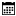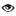s

#### Sample Programs

Murugan Andezuthu Dharmaratnam |01 March 2021 |4589

Here we will write an 8086 assembly language program to input a 5 digit number from the keyboard and display the sum of the digits. This program can be broken into 2 main sections.

1. Read a 5 digit number with validation to check if the entered character is numeric. If the entered character is valid add to a dw sum
2. Convert the numeric sum into ASCII so that it can be displayed

## Code To calculate the sum of 5 digit number

```.model tiny
.code

org 100h

main proc near

; here is the section to read a valid 5 digit number and keep adding to dw sum
mov ah,09h  ; dos function 09h to display a string
mov dx,offset messagereadnumber ; offset of message
int 21h     ; dos interrupt 21h

mov sum,0
mov cx,5    ; cx is set to 5 so that it loops 5 times to enter 5 digits of the number
push cx

mov ah,01h  ; read character from input store result in al
int 21h     ; dos interrupt 21h

; code to check if the number is between 0 and 9
cmp al,30h  ; 30h is character 0
jl invalidnumber    ; If the number is less than character 0 jump to invalidnumber

cmp al,39h  ; 39h is character 9
jg invalidnumber    ; if the number is greater that character 9 jump to invalid number
; end code to check if the number is between 0 and 9

sub al,30h  ; 30h is the hex code for character 9 you subtract 30h to get the number and not character
mov ah,00

pop cx

jmp validnumber

invalidnumber:
mov ah,09h
mov dx,offset messageinvalidnumber
int 21h

; end section to read a valid 5 digit number

validnumber:
mov ax,sum

; code to convert decimal number to asii
mov si,offset numbertoascii
mov cx,00h
mov bx,0ah
hexloop1:
mov dx,0
div bx
push dx
inc cx
cmp ax,0ah
jge hexloop1
mov [si],al
hexloop2:
pop ax
inc si
mov [si],al
loop hexloop2
inc si
mov [si],'\$'
; end convert decimal number to asii

mov ah,09h  ; dos function to display a string
mov dx,offset displayresult ; offset of message to display since I am not terminating
; the string displayresult with a dollar it will print
; the displayresult also which terminates with a \$
int 21h     ; dos interrupt 21h

mov al,00
int 21h     ; dos interrupt 21h

endp
messageinvalidnumber db 0ah,0dh,"Invalid number\$"